# Confusion in the derivation of the force on a magnetic dipole

## Homework Statement

ok this is not a homework but i am confused by the derivation of ## f = \nabla (m . B)##
both start out with ##\vec B(r) \approx \vec B_0 + (\vec r' . \nabla)\vec B|_0 ... \\
f = \iiint_{v'} \vec J( r') \times (\vec r' . \nabla)\vec B|_0
##
where prime is body coordinates and unprime is space coordinates
the first one uses vector identities
##
(\vec r' . \nabla)\vec B = \nabla (\vec r' . B) - r' \times (\nabla \times B)
##
the other two terms die out because of different coordinates
then it proceeds to say curl of magnetic field is zero about the origin if it is due to external sources
then the proof proceeds i have posted in the photo

the second proof(from girffiths) uses le cevitas and kronecker deltas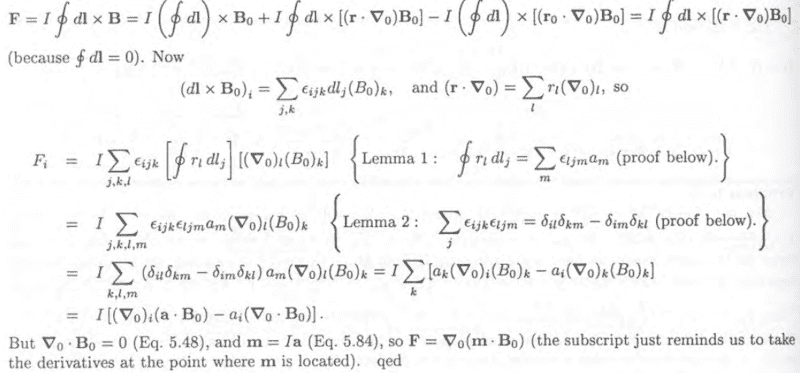while both seem to arrive at the same answer of ## f = \nabla(\vec m . B)## after this griffiths specifically mentions that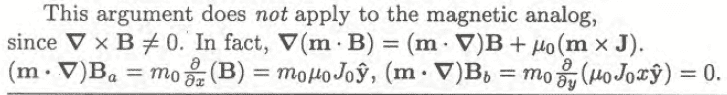he mentions that ##\nabla \times B \neq 0##
honestly to me the first proof makes more sense because the magnetic field used is due to external sources and should not have curl at the origin but questioning seems griffiths seems inplausible so
what actually is correct sorry for the long post it has been bugging me for a long while now

## The Attempt at a Solution

#### Attachments

TSny
Homework Helper
Gold Member
while both seem to arrive at the same answer of ## f = \nabla(\vec m . B)## after this griffiths specifically mentions that View attachment 231421
he mentions that ##\nabla \times B \neq 0##
honestly to me the first proof makes more sense because the magnetic field used is due to external sources and should not have curl at the origin but questioning seems griffiths seems inplausible so
what actually is correct sorry for the long post it has been bugging me for a long while now
Both proofs end up with ##\vec F = \vec \nabla (\vec m \cdot \vec B )##. The first proof assumed ##\vec \nabla \times \vec B = 0## at the location of the dipole while Griffiths' proof did not assume this. So, Griffiths has shown that the result holds even if the dipole is located at a point where the external field does not satisfy ##\vec \nabla \times \vec B = 0##.

Griffiths gives a specific example where ##\vec \nabla \times \vec B \neq 0## at the location of the dipole ##\vec m##. This is problem 6.5 in the 3rd edition of the text. The dipole is immersed in a block of material for which there is a uniform current density ##\vec J## in the material. This current density produces the “external” field felt by the dipole. So, in this case, ##\vec \nabla \times \vec B \neq 0## at the location of the dipole. Instead, ##\vec \nabla \times \vec B = \mu_0 \vec J## . But Griffiths' proof is still applicable to this situation. So you can still conclude that for this example you would have ##\vec F = \vec \nabla (\vec m \cdot \vec B)##.

You quote Griffiths as follows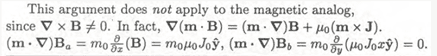To understand this, you need to see the full context.
This is related to part (c) of problem 6.5, which states

“In the electrostatic case the expressions ##\vec F = \vec \nabla (\vec p \cdot \vec E)## and ##\vec F = (\vec p \cdot \vec \nabla ) \vec E## are equivalent (prove it), but this is not the case for the magnetic analogs (explain why).”

Griffiths’ solution for this part contains the following,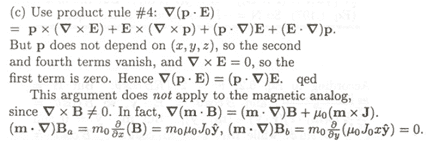[The very last line in this quote applies to the specifics of parts (a) and (b) of problem 6.5.]

#### Attachments

•timetraveller123
oh i see now it now

so griffiths is not saying that
curl of B is due to the dipole itself but only when there is external current density through the dipole
thats what was confusing me

since they both arrive at same result is there any way to continue the first proof without assuming curl is zero?

TSny
Homework Helper
Gold Member
oh i see now it now

so griffiths is not saying that
curl of B is due to the dipole itself but only when there is external current density through the dipole
Yes.

since they both arrive at same result is there any way to continue the first proof without assuming curl is zero?
Yes, I believe so. Look at the following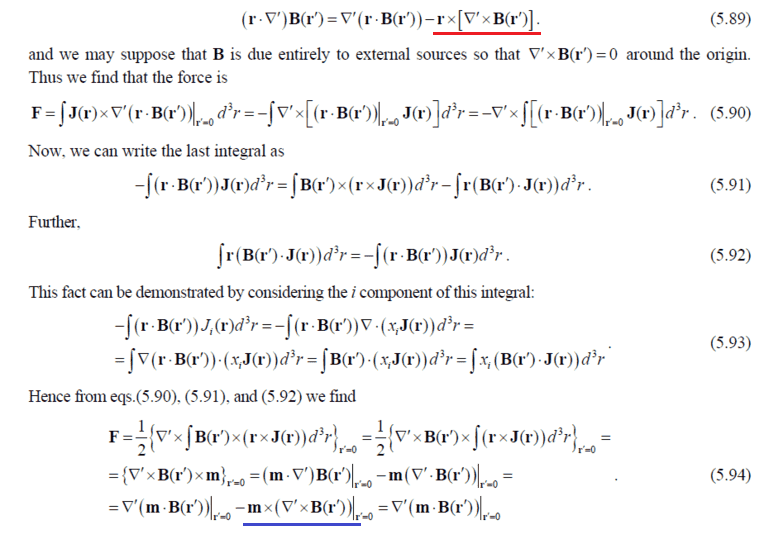The term underlined in red in (5.89) will not be zero if the curl of B is nonzero. This will lead to an extra term in 5.90 which can be shown (using various manipulations along with ##\vec \nabla \cdot \vec J = 0) ## to equal ##\vec m \times (\vec \nabla ' \times \vec B(\vec{r}') ##. But this is exactly what is needed to cancel the term underlined in blue in the last line of (5.94). So you still get ##\vec F = \vec \nabla ' \left( \vec m \cdot \vec B(\vec {r}') \right)|_{r' = 0} ## without having to assume the curl of B is zero.

#### Attachments

•timetraveller123
woah thats insane i will take a look at it tmr right now its 11pm where i am now i will try to derive what you said tmr morning thanks for the help and sorry for late reply

sorry for the very late reply i was quite busy
so i tried to prove what you said and i couldn't really do it could you give me head start thanks

TSny
Homework Helper
Gold Member
so i tried to prove what you said and i couldn't really do it could you give me head start thanks
The term underlined in red in post #4 leads to an extra contribution to the force ##\vec F## of equation (5.90) of the form

##\vec {F}_{\rm extra} = -\int \vec J(\vec r) \times \left[\vec r \times \left( \vec {\nabla}' \times \vec B(\vec r') \right) \bigg|_{r'=0} \right] d^3r##

For convenience, let ##\vec S \equiv \left( \vec {\nabla}' \times \vec B(\vec r') \right) \bigg|_{r'=0}##.

So, ##~~\vec {F}_{\rm extra} = -\int \vec J(\vec r) \times \left(\vec r \times \vec S \right) d^3r##

Use a vector identity to show

##\vec {F}_{\rm extra} = -\left[\int \vec J(\vec r) \cdot \vec r d^3r \right] \vec S + \int \left( \vec J(\vec r) \cdot \vec S\right) \vec r d^3r##

EDIT: The signs of both terms on the right are wrong. It should read ##\vec {F}_{\rm extra} = \left[\int \vec J(\vec r) \cdot \vec r d^3r \right] \vec S - \int \left( \vec J(\vec r) \cdot \vec S\right) \vec r d^3r##

The integral in the first term on the right can be shown to be zero. In order not to lose the train of thought, we can come back later to prove it. So, accepting it for now, we have

##\vec {F}_{\rm extra} = \int \left( \vec J(\vec r) \cdot \vec S\right) \vec r d^3r##

You want to show that this will cancel the term underlined in blue in (5.94) of post #4. That is, you need to show that

##\vec m \times \left( \vec {\nabla}' \times \vec B(\vec r') \right) \bigg|_{r'=0} = \int \left( \vec J(\vec r) \cdot \vec S\right) \vec r d^3r ##

Recalling the definition of ##\vec S##, you need to show

##\vec m \times \vec S = \int \left( \vec J(\vec r) \cdot \vec S\right) \vec r d^3r ##

This requires some additional manipulations and use of the identity given in equation (5.92) except with ##\vec B(\vec r')## in (5.92) replaced by ##\vec S## .

Last edited:
ok thanks
actually thats what i did at first but i was trying to hopelessly prove the second term was zero

for the second term
##
(\int (J \cdot S)r d^3 r)_i = \sum_j S_j \int J_J r_i d^3 r = \sum_j S_j \int \nabla \cdot (r_j J) r_i d^3 r -\sum_j S_j \int (r_j J)\cdot \nabla(r_i) d^3 r \\
(\int (J \cdot S)r d^3 r)_i = -\sum_j S_j \int r_j J_i d^3 r\\
\int (J \cdot S)r d^3 r = -\int J (r \cdot S) d^3 r\\

\int S \times (r \times J) d^3 r = \int r (S \cdot J ) - J(S \cdot r) d^3 r\\
S \times m = \int r (S \cdot J) d^3 r
##
why am i missing a negative sign?????

for proving the first term to be zero i am not too sure but this is my guess
##
\int ( \mu_0J\cdot r) d^3 r = \int (\nabla \cdot (B(r) \times r)) - B(r) \cdot( \nabla \times r) d^3r
##
both the terms on the right go to zero hence the integral is zero is this correct?

Last edited:
TSny
Homework Helper
Gold Member
why am i missing a negative sign?????
You're not missing a negative sign. It's my mistake. Sorry about that. I wrote in post #7
Use a vector identity to show

##\vec {F}_{\rm extra} = -\left[\int \vec J(\vec r) \cdot \vec r d^3r \right] \vec S## + ##\int \left( \vec J(\vec r) \cdot \vec S\right) \vec r d^3r##
The signs of both terms on the right are wrong.

for proving the first term to be zero i am not too sure but this is my guess
##
\int ( \mu_0J\cdot r) d^3 r = \int (\nabla \cdot (B(r) \times r)) - B(r) \cdot( \nabla \times r) d^3r
##
both the terms on the right go to zero hence the integral is zero is this correct?
Yes. That looks good to me.

You used ##\mu_0 \vec J = \vec {\nabla} \times \vec B##, so you are assuming that there are no time-dependent electric fields present. I noticed that Jackson derives ##\vec F = \vec {\nabla} (\vec m \cdot \vec B) ## and states that, "This result holds even for time-varying external fields." But he does appear to assume steady currents so that ##\vec {\nabla} \cdot \vec J = 0##.

I believe that you can show that ##\int \vec J(\vec r) \cdot \vec r d^3r = 0## just using ##\vec {\nabla} \cdot \vec J = 0##. So, I think the "first proof" of your first post can be generalized to cases where ##\vec {\nabla} \times \vec B = \mu_0 \vec J + \mu_0 \varepsilon_0 \frac{\partial \vec E}{\partial t}## as long as ##\vec {\nabla} \cdot \vec J = 0##.

Last edited:
Homework Helper
Gold Member
You can do these lengthy vector algebra proofs, but the way I like to look at is that you can bring the dipole in from infinity if you have a field pointing in one direction, and turn the magnetic dipole perpendicular to the field so that it experiences zero force and zero torque. Then when you get to the location of interest, you rotate it and find ## U=-m \cdot B ## because the torque ## \tau=m \times B ## has a ## \sin{\theta} ## in the cross product, and integrated over ## \theta ## becomes a cosine or dot product. Perhaps this is a handwaving argument, but I find it satisfactory to explain the energy term. Meanwhile ## F=-\nabla U ##.

TSny
Homework Helper
Gold Member
You can do these lengthy vector algebra proofs, but the way I like to look at is that you can bring the dipole in from infinity if you have a field pointing in one direction, and turn the magnetic dipole perpendicular to the field so that it experiences zero force and zero torque. Then when you get to the location of interest, you rotate it and find ## U=-m \cdot B ## because the torque ## \tau=m \times B ## has a ## \sin{\theta} ## in the cross product, and integrated over ## \theta ## becomes a cosine or dot product. Perhaps this is a handwaving argument, but I find it satisfactory to explain the energy term. Meanwhile ## F=-\nabla U ##.
Hey, Charles. Yes, it would seem that your approach gets to the result quickly. But, apparently, there are some subtleties to this topic. Jackson points out that ## U=-m \cdot B ## should not be interpreted as the total potential energy of the magnetic moment in the external B field. According to him, you do need to worry about the process of bringing the dipole to its final location. I won't try to quote him here unless you want me to. I noticed that Jackson made some significant changes in some of his remarks about the force on a magnetic dipole when he went from the 2nd to the 3rd edition. If you have access to the American Journal of Physics you can find a number of papers on the topic (some fairly recent).

•Homework Helper
Gold Member
Hey, Charles. Yes, it would seem that your approach gets to the result quickly. But, apparently, there are some subtleties to this topic. Jackson points out that ## U=-m \cdot B ## should not be interpreted as the total potential energy of the magnetic moment in the external B field. According to him, you do need to worry about the process of bringing the dipole to its final location. I won't try to quote him here unless you want me to. I noticed that Jackson made some significant changes in some of his remarks about the force on a magnetic dipole when he went from the 2nd to the 3rd edition. If you have access to the American Journal of Physics you can find a number of papers on the topic (some fairly recent).
I think the problem also gets more complex when the magnetic dipoles are the result of the response of the material to the magnetic field, which is in some cases results in the magnetic moments experiencing the magnetic field that they helped to create. It appears to be a non-trivial problem. If I get some spare time, I may study it further.

sorry for the late reply was quite busy again

i thought ## u = -m\cdot B## was derived from the derived from the force equation not the other way around

@TSny
i dont understand why would there be time varying electric field because the B and J in the equations are due to the magnetic dipole itself and if the current is steady then why would there be time varying electric field

Homework Helper
Gold Member
i thought u=−m⋅Bu=−m⋅B u = -m\cdot B was derived from the derived from the force equation not the other way around
## U=-m \cdot B ## comes from the torque equation, instead of the force equation. It is integrated over ## \theta ##.

from what i understand
##
\nabla U = - f = -\nabla(m \cdot B)
##
hence the equation i always thought the toque way of deriving was not rigorous

•Homework Helper
Gold Member
from what i understand
##
\nabla U = - f = -\nabla(m \cdot B)
##
hence the equation i always thought the toque way of deriving was not rigorous
That's probably correct. That's what @TSny has also said in post 11 above. I need to study the finer details of the more rigorous derivations, but so far, I haven't yet found the time.

TSny
Homework Helper
Gold Member
@TSny
i dont understand why would there be time varying electric field because the B and J in the equations are due to the magnetic dipole itself and if the current is steady then why would there be time varying electric field
I believe B is the field due to external sources. B is not the field produced by the currents of the magnetic dipole itself. Jackson gives a derivation of ##\vec F = \vec {\nabla} (\vec m \cdot \vec B)## where ##\vec m## is due to a localized distribution of current ##\vec J## and it is assumed that the current density of the magnetic dipole obeys ##\vec {\nabla} \times \vec J = 0## [Edit: This should be ##\vec {\nabla} \cdot \vec J = 0##] . In the second edition of his text, Jackson states that the result of the derivation "holds even for time-varying external fields." I interpreted this to mean that the external B could be time-dependent. A time-dependent B is always accompanied by an electric field E obeying ##\vec {\nabla} \times \vec E = - \frac{\partial{\vec B}}{\partial t}##. In general, ##\vec E## could be time-dependent, too.

However, I later looked at Jackson's 3rd edition. He gives the same derivation of ##\vec F = \vec {\nabla} (\vec m \cdot \vec B)##, but he drops the remark that I quoted above. Instead, he adds the following comments which I find confusing: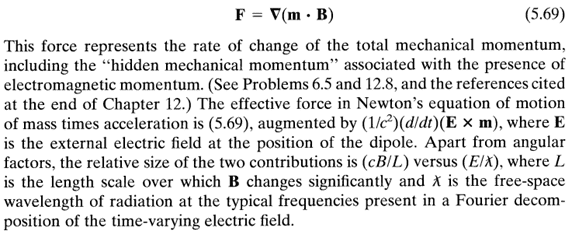I then decided to browse through the American Journal of Physics for articles related to this. I found a number of articles which I only skimmed through. This is when I decided that when there are time-dependent external fields, there are subtleties to this topic that would require quite a bit of time to sort out (for me, anyway).

#### Attachments

Last edited:
•but in my case the term which i was supposed to prove zero was
##
\int J(r)\cdot r d^3 r
##
the J is the body current and not space current and according when using the ampere law shouldn't the magnetic field refer to that of the body(dipole) and not space magnetic field

Homework Helper
Gold Member
mm→\vec m is due to a localized distribution of current →JJ→\vec J and it is assumed that the current density of the magnetic dipole obeys →∇×→J=0∇→×J→=0\vec {\nabla} \times \vec J = 0.
@TSny (From post 17) You are saying ## \nabla \times J=0 ##. ## \\ ## Do you mean ## \nabla \cdot J=0 ## ? For a magnetic dipole, ## J ## moves around in a circle or other loop, so that ## \nabla \times J \neq 0 ##. Alternatively, we can apply Stokes theorem around the loop of the dipole, and clearly ## \oint J \cdot dl \neq 0 ##. ## \\ ## I believe it should say ## \nabla \cdot J=0 ##.

TSny
Homework Helper
Gold Member
@TSny (From post 17) You are saying ## \nabla \times J=0 ##. ## \\ ## Do you mean ## \nabla \cdot J=0 ## ? For a magnetic dipole, ## J ## moves around in a circle or other loop, so that ## \nabla \times J \neq 0 ##. Alternatively, we can apply Stokes theorem around the loop of the dipole, and clearly ## \oint J \cdot dl \neq 0 ##. ## \\ ## I believe it should say ## \nabla \cdot J=0 ##.
Yes, thanks for the correction.

•TSny
Homework Helper
Gold Member
but in my case the term which i was supposed to prove zero was
##
\int J(r)\cdot r d^3 r
##
the J is the body current and not space current and according when using the ampere law shouldn't the magnetic field refer to that of the body(dipole) and not space magnetic field
Yes. You showed ##\int \vec{J}(r) \cdot \vec r d^3 r = 0## in post #8 where you wrote
##
\int ( \mu_0J\cdot r) d^3 r = \int (\nabla \cdot (B(r) \times r)) - B(r) \cdot( \nabla \times r) d^3r
##
I believe the sign of the last term is wrong, but it doesn't matter. Here, B(r) is the magnetic field produced by the body current; that is, the current that's producing the dipole moment. Nevertheless, in the attachment of your first post, B(r') is the space field; that is, the field produced by external sources. I don't see any problem.

•timetraveller123
I believe the sign of the last term is wrong, but it doesn't matter. Here, B(r) is the magnetic field produced by the body current; that is, the current that's producing the dipole moment. Nevertheless, in the attachment of your first post, B(r') is the space field; that is, the field produced by external sources. I don't see any problem.
i dont quite get your point

i was trying to respond to your comment that jackson shows that the result holds even in time varying electric fields
In the second edition of his text, Jackson states that the result of the derivation "holds even for time-varying external fields." I interpreted this to mean that the external B could be time-dependent. A time-dependent B is always accompanied by an electric field E obeying →∇×→E=−∂→B∂t∇→×E→=−∂B→∂t\vec {\nabla} \times \vec E = - \frac{\partial{\vec B}}{\partial t}. In general, →EE→\vec E could be time-dependent, too.

what i quite confused about is that all the fields and current concerning proving the term zero only pertains to those of the body and not space and if the dipole has a steady current then why is there changing electric field

TSny
Homework Helper
Gold Member
what i quite confused about is that all the fields and current concerning proving the term zero only pertains to those of the body and not space and if the dipole has a steady current then why is there changing electric field
The dipole will not produce a changing electric field since the current density of the dipole is assumed to be steady. But the dipole could nevertheless be acted on by externally applied E and B fields that vary in time. In Jackson's 2nd edition, it is claimed that the result ##\vec F = \vec \nabla ( \vec m \cdot \vec B )## holds even when you have such time-varying applied fields. However, as mentioned earlier, Jackson modifies this claim in the 3rd edition. See post #17. The correct handling of time-varying applied fields appears to be complicated.

Your proof of ##\int \vec r \cdot \vec J(\vec r) d^3 r = 0## seems to me to be valid. It has nothing to do with the externally applied fields. You only needed to use ##\vec \nabla \times \vec B_{\rm dipole} = \mu_0 \vec J##. Here ##\vec J## is the current density producing the dipole ##\vec m## and ##\vec B_{\rm dipole}## is the magnetic field produced by ##\vec J##.

oh see what you mean now

looking i am realizing that there is a major flaw in my proof for that term being zero
S is a function of space coordinates r' and not r
so infact
##
s(r') = \mu_0J(r') + \mu_0 \epsilon_0 \frac {\partial E(r')}{\partial t}
##
i think this is where the time varying applied fields come in
so how to prove this term to be zero

TSny
Homework Helper
Gold Member
Here is a way that I believe you can show ##\int \vec r \cdot \vec J(\vec r) d^3r = 0## just using the fact that the current density is steady, so ##\vec \nabla \cdot \vec J = 0##.

Let ##x_i## be the ##i##th component of ##\vec r##, where ##\vec r## is the argument of ##\vec J(\vec r)##. The index ##i## could be 1, 2, or 3.

Using a vector identity, we have

##\vec \nabla \cdot \left( x_i^2 \vec J(\vec r) \right) = \vec J(\vec r) \cdot \vec \nabla(x_i^2) + x_i^2 \vec \nabla \cdot \vec J = \vec J(\vec r) \cdot \vec \nabla(x_i^2) = 2 x_i J_i(\vec r) \,\,\,\,\,\,\, (\text{ no sum over i})##

Integrate both sides over all space and use the divergence theorem to show that the integral of the left side is zero.

So, ##\int x_i J_i(\vec r) d^3r = 0## for each ##i = 1, 2, 3##.

Therefore, ##0 = \sum \limits_{i = 1}^3 \int x_i J_i(\vec r) d^3r = \int \vec r \cdot \vec J(\vec r) d^3r## .

•wait nevermind ignore my last post i got confused
∇⋅(x2i→J(→r))=→J(→r)⋅→∇(x2i)+x2i→∇⋅→J=→J(→r)⋅→∇(x2i)=2xiJi(→r)( no sum over i)∇→⋅(xi2J→(r→))=J→(r→)⋅∇→(xi2)+xi2∇→⋅J→=J→(r→)⋅∇→(xi2)=2xiJi(r→)( no sum over i)\vec \nabla \cdot \left( x_i^2 \vec J(\vec r) \right) = \vec J(\vec r) \cdot \vec \nabla(x_i^2) + x_i^2 \vec \nabla \cdot \vec J = \vec J(\vec r) \cdot \vec \nabla(x_i^2) = 2 x_i J_i(\vec r) \,\,\,\,\,\,\, (\text{ no sum over i})
oh wow thats very smart i was trying to look for something along that line i believe
##
\int J d^3r = 0
##
is proved in a similar way

thanks for the help i have a side question
is this
##
\vec c \cdot (\nabla \vec d)
##
same as
##
(\vec c \cdot \nabla) \vec d
##

from what i understand gradient of vectors gives tensors

TSny
Homework Helper
Gold Member
wait nevermind ignore my last post i got confused

oh wow thats very smart i was trying to look for something along that line i believe
##
\int J d^3r = 0
##
is proved in a similar way
Yes. I had seen this way of proving ##\int \vec J d^3r = 0##, which helped in constructing the proof of ##\int \vec r \cdot \vec J d^3r=0##.

thanks for the help i have a side question
is this
##
\vec c \cdot (\nabla \vec d)
##
same as
##
(\vec c \cdot \nabla) \vec d
##
Yes, if you interpret the notation ##\nabla \vec d## as a dyadic quantity, then I believe the two expressions are the same. A dyadic is a tensor quantity. See https://en.wikipedia.org/wiki/Dyadics

•timetraveller123
thanks one last question

in geometric calculus (have no idea what it is) i saw gradient of a vector function f defined as
divergence of f plus the curl(or something like that) f
why is there such a definition it does not make sense dimensionally the first is a scalar and the second is i am guessing higher order than vector
•